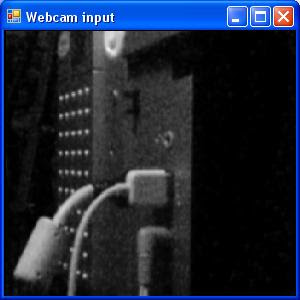## Thursday, March 11, 2010

### Some basic image processing operations with F#

The previous post presented a way to access the image data from the Webcam using DirectShow.Net and F#. We can manipulate this data to do some basic image processing operations with it.

#### Converting image to gray scale

Many image processing operations and algorithms are defined for grayscale images. A simple way to convert the image to grayscale is the following:

``let grayGrabber(transform) =      fun (width,height) ->      {  new ISampleGrabberCB with             member this.SampleCB(sampleTime:double , pSample:IMediaSample )= 0                                  member this.BufferCB(sampleTime:double , pBuffer:System.IntPtr , bufferLen:int) =                                       let grayImage = getGrayImage pBuffer bufferLen                                                let resultImage = transform width height grayImage                                saveGrayImageToRGBBuffer resultImage  pBuffer bufferLen                                                                                   0                }``

Given that we have:

``let inline getGrayImage (data:IntPtr) (size:int)  =     let grayImage = Array.create (size/3) (byte(0))    let pixelBuffer = Array.create 3 (byte(0))    let mutable it = 0    for i in 0..(size - 3 ) do        if (i + 1) % 3 = 0 then           Marshal.Copy(new System.IntPtr(data.ToInt32()+i),pixelBuffer,0,3) |> ignore          grayImage.[it] <-  byte(float(pixelBuffer.)*0.3 +                                   float(pixelBuffer.)*0.59 +                                   float(pixelBuffer.)*0.11)          it <- it+1    grayImagelet inline saveGrayImageToRGBBuffer (grayImage:byte array) (data:IntPtr) (size:int) =    let mutable targetIndex = 0    for i in 0..(size/3 - 1) do      let p = grayImage.[i]      Marshal.WriteByte(data,targetIndex,p)      Marshal.WriteByte(data,targetIndex+1,p)      Marshal.WriteByte(data,targetIndex+2,p)      targetIndex <- targetIndex+3      ()``

The technique for converting the image to grayscale was taken from here.

Using this new grabber definition we can change the code from the previous post to do this:

``let mediaControl,filterGraph = createVideoCaptureWithSampleGrabber                                       device                                       nullGrayGrabber                                      None``

Given that `nullGrayGrabber` is defined as:

``let nullGrayGrabber = grayGrabber (fun (_:int) (_:int) image -> image)``

The original webcam output looks like this:By applying this filter the webcam output looks like this:#### Applying templates

With this definitions we can do a common operation in image processing called template convolution. Basically, this technique consists in applying an operation to each pixel of an image and its surrounding neighborhood . The operation is represented by a matrix, for example:

``0.0  1.0 0.01.0 -1.0 1.00.0  1.0 0.0``

To apply this template to the a pixel of the image at x',y' we write:

``(0.0*image[x-1,y-1]) + (1.0*image[x,y-1]) + (0.0*image[x+1,y-1]) +(1.0*image[x-1,y])   + (-1.0*image[x,y]) +  (1.0*image[x+1,y]) +(0.0*image[x-1,y+1]) + (1.0*image[x,y+1]) + (0.0*image[x+1,y+1]) ``

The function to apply this kind of operation looks like this:

``let convolveGray3 w h (image:byte array) (template:float[,]) hhalf whalf =         let result = Array.create (image.Length) (byte(0))      let getPixelGray' = getPixelGray w h image   let setPixelGray' = setPixelGray w h result      for y in (hhalf + 1) .. (h - ((Array2D.length1 template) - hhalf - 1) - 1 ) do       for x in (whalf + 1) .. (w - ((Array2D.length2 template) - whalf - 1) - 1 ) do                    let mutable r = 0.0                    for ty in 0 .. (Array2D.length1 template - 1) do          for tx in 0 .. (Array2D.length2 template - 1) do             let ir = getPixelGray' ( x + (tx - whalf)) ( y + (ty - hhalf))             r <- r + template.[ty ,tx ]*float(ir)                         setPixelGray' x y (byte(Math.Abs r))         result``

Given that:

``let inline getPixelGray width height (image:byte array) x y  =   let baseHeightOffset = y*width   let offset = baseHeightOffset + x   image.[offset]let inline setPixelGray width height (image:byte array) x y value1 =   let baseHeightOffset = y*width   let offset = baseHeightOffset + x   image.[offset] <- value1     ()``

We can represent operations such as edge detection or smoothing.
First order edge detection can be represented using the following template:

``let firstOrderEdgeDetectTemplate =              (array2D [|[|2.0;-1.0|];                        [|-1.0;0.0|];|])   ``

Changing the code of the main program to the following:

``let convGrayGrabber1 =     grayGrabber (fun width height grayImage -> convolveGray3 width height grayImage firstOrderEdgeDetectTemplate 0 0 ) ``

Now the output looks like:We can also use a template template to represent averaging. This technique removes detail from the image by calculating the average value of pixel given its neighborhood. The definition of a function:

``let averagingTemplate (windowSize) =  Array2D.create windowSize windowSize (1.0/float(windowSize*windowSize))``

This function creates a template which looks like this:

``> averagingTemplate 3;;val it : float [,] = [[0.1111111111; 0.1111111111; 0.1111111111]                      [0.1111111111; 0.1111111111; 0.1111111111]                      [0.1111111111; 0.1111111111; 0.1111111111]]``

We can change again the main program to use this function:

``let averagingGrayGrabber =     let template = averagingTemplate 3    grayGrabber (fun width height grayImage -> convolveGray3 width height grayImage template 1 1 )...let mediaControl,filterGraph = createVideoCaptureWithSampleGrabber                                       device                                       averagingGrayGrabber                                       None``

The result image looks like this:#### Final words

As with the previous post I'm using mainly the imperative features of F# . For future posts I'll try change this and also continue the exploration of image processing with F#.

Most of the techniques described here were taken from the "Feature Extraction & Image Processing" book by Mark S. Nixon and Alberto S. Aguado.

Code for this post can be found here.